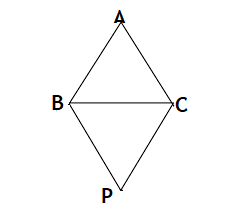Maths / Areas Of Parallelograms And Triangles / Area of Triangle

QUESTION

In the given figure, if ar(PBC) = 20 sq units, then ar(ABPC) = ________________OPTIONS A. 20 sq units B. 40 sq units C. 30sq units D. 80 sq. units
Right Option : B

EXPLANATION
Explain TypeExplanation Content
Text

ar(ABPC) = 20 + 20 = 40 sq units as both triangles are congruent and congruent triangles have equal area.

TestimonialsSTUDENT FEEDBACK - Ayan ghosh C/o ABHYAS Academy
8th
My experience was very good with Abhyas academy. I am studying here from 6th class and I am satisfied by its results in my life. I improved a lot here ahead of school syllabus.